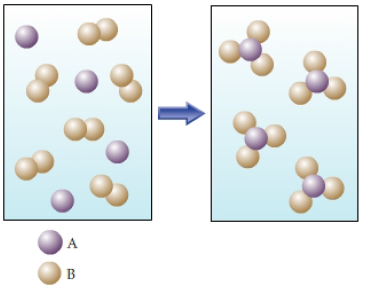Problem: Part A. The figure shows the reaction of element A (lavender spheres) with element B (tan spheres). Write the balanced chemical equation for this reaction in terms of A and B.Express your answer as a chemical equation.When a chemical reaction occurs, atoms rearrange to form new compounds, but no new atoms are created nor are any destroyed. This concept is called conservation of mass. Mass conservation can be seen in a balanced chemical equation, where the numbers of each kind of atom are the same on both sides of the reaction arrow.

FREE Expert Solution
80% (143 ratings)Problem Details

Part A. The figure shows the reaction of element A (lavender spheres) with element B (tan spheres). Write the balanced chemical equation for this reaction in terms of A and B.When a chemical reaction occurs, atoms rearrange to form new compounds, but no new atoms are created nor are any destroyed. This concept is called conservation of mass. Mass conservation can be seen in a balanced chemical equation, where the numbers of each kind of atom are the same on both sides of the reaction arrow.